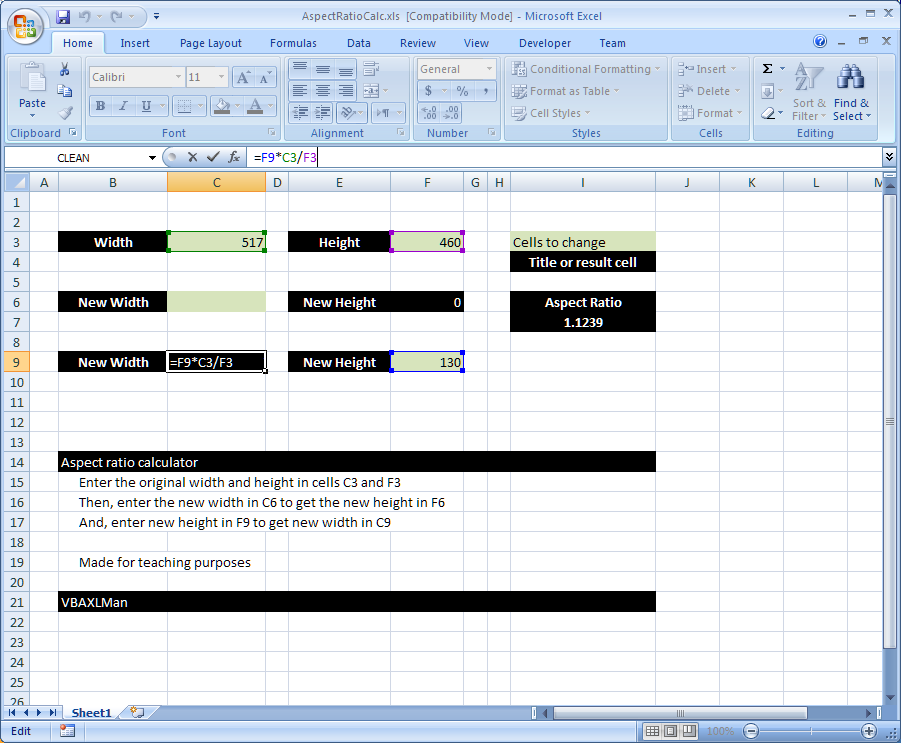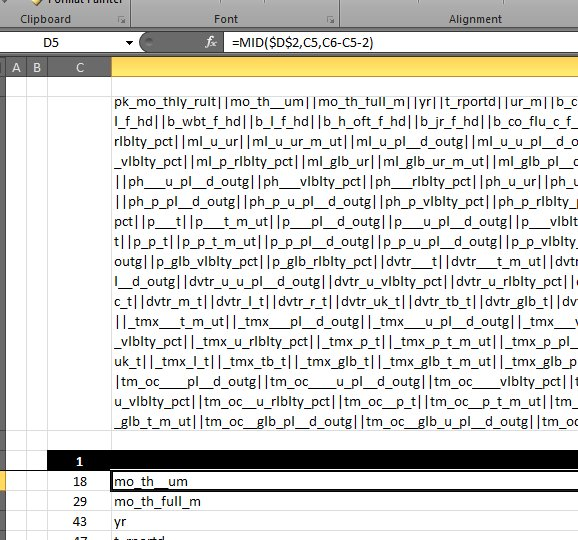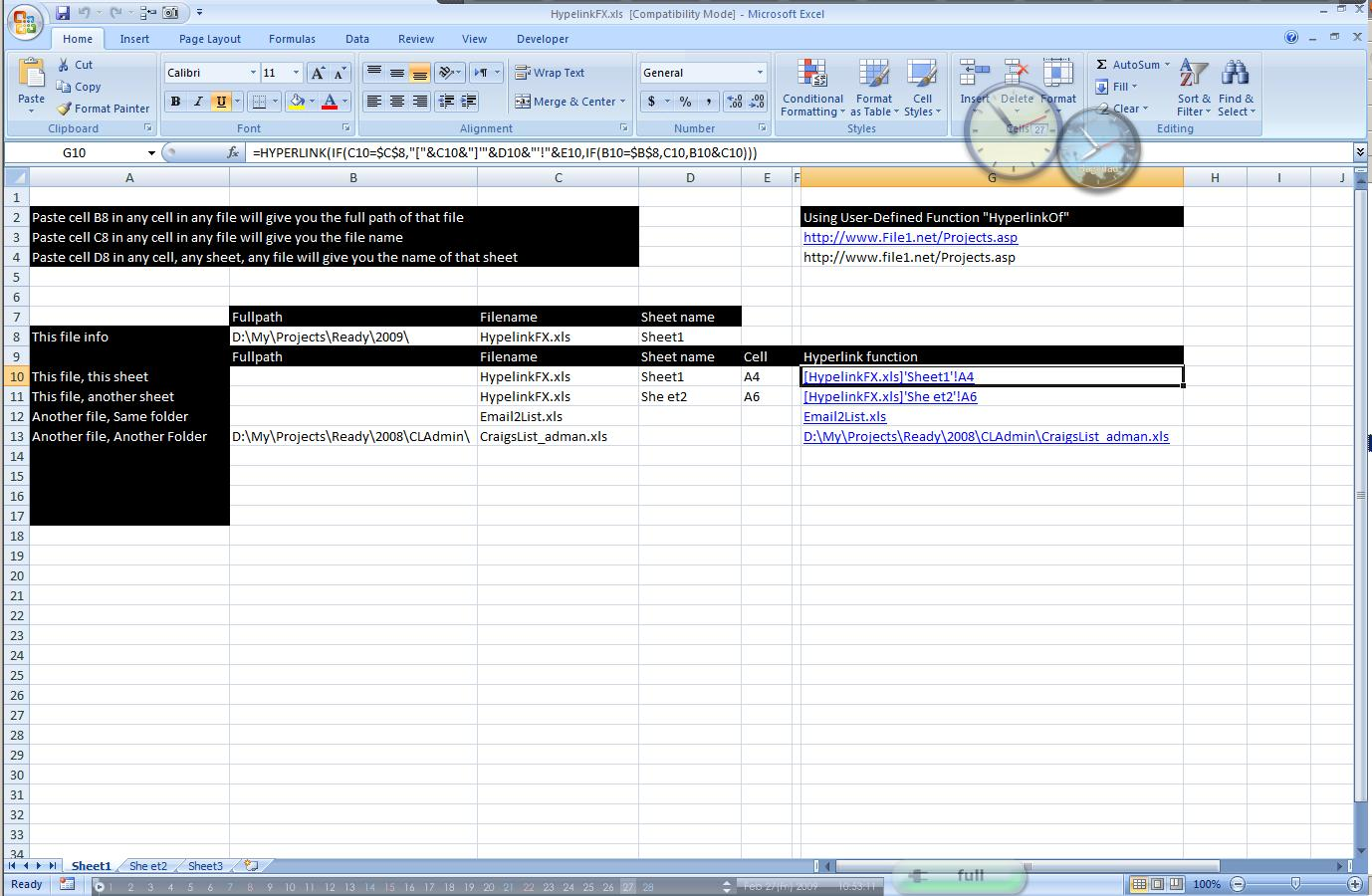## Standard math to calculate Aspect RatioHere is a simple formula that uses standard math functions (+ , – , / and *) to calculate the aspect ratio I often need some simple formula like this …

## MID + SEARCH to convert cell to rowsI have been there and done that more than once before This time I had a really large cell, more than 3k characters need to be converted into rows, and …